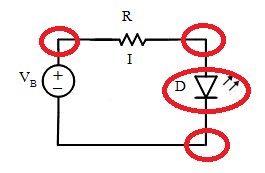# Simulate, Analyze, Measure Electronics (SAME)

In Electronics, there are 3 perspectives of a circuit

1. Simulation - simulate to observe what the real world results should be like
2. Analysis - using mathematical models to analyze and predict the results
3. Measurement - prototype the circuit and measure
If the results from the 3 perspectives are the SAME, one can independently conclude that the result is correct. This is a good way to learn electronics and indispensable when one needs to modify an existing circuit or design a new circuit.

## We like short equations

An equation with more than 2 fingers width on each side of the equation is not very useful in design
Rinaldo Graziadei

The voltage gain of a common emitter amplifer can be written asor this shorter version

\begin{equation} A_V = -{R_C \over r_e} = -{{R_C I_E} \over 26mV } \end{equation}

On this site, we prefer the shorter one - because it enables the reader to see quickly that the main determinants of the voltage gain are the parameters RC and IE. This is the first order effect. The long equation is more useful for higher order analysis.

## The odds are against you in the lab

The odds are overwhelmingly against the learner to get a new circuit working right the first time
Dr GH TanThe circuit shown is a simple LED circuit. The circled parts of the circuits are the places where the learner has to wire or orientate the part correctly. Lets say that there is a 1/2 chance of getting each action done right. The circuit works if and only if all the actions are done correctly and the probability of that happening is

\begin{equation} P = {1 \over 2} \times {1 \over 2} \times {1 \over 2} \times{1 \over 2} = {1 \over 16} \end{equation}

So you have actually better odds guessing the outcome of a coin toss then getting this 'simple' circuit wired up correctly the first time you attempt it.

We improve the odds by

• using a breadboard simulator to check the wiring.
• using basic theory to help you predict actual circuit results.
• using online simulation to show the user the outcome of a correct circuit operation.

## Start with the end in mind

Although in the do-re-mi song, we are told to start from the very beginning - in practice, it is not always a good place to start. Starting with the end in mind actually provides the learner with purpose. Try it with our projects like the 24 hour clock circuit. In this approach, you only learn what is required to solve the problem on hand.

## How we can help you

• For students, you are welcome to seek clarification on what we have presented. Sorry, we don't do homework. Also do not just copy the results from our simulators but rather work out your solution and use the simulator output to check. Anything worth learning requires effort. If you find any lesson useful, please recommend it to your friends.
• For lecturers, let us help you build the scaffolds you need for the students in your blended classroom. Tell us what you want in your flipped classroom for electronics. If you find this site useful, please use it as a reference for your students.

Looking forward to your comments.

## Work in Progress

We are a work in progress. We are not done yet

With advertising revenues falling despite increasing numbers of visitors, we need your help to maintain and improve this site, which takes time, money and hard work. Thanks to the generosity of our visitors who gave earlier, you are able to use this site at no charge.

If you have benefited from this site and are able, please give \$10 via Paypal. It will allow us continue into the future. It only takes a minute. Thanks!